### Home > CALC > Chapter 11 > Lesson 11.2.3 > Problem11-73

11-73.
1.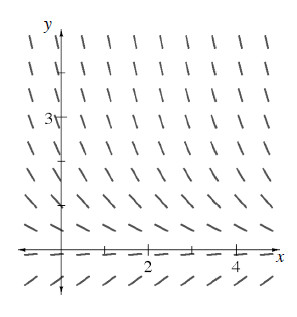Multiple Choice: The slope field shown at right is the graph of: Homework Help ✎

1.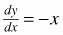2.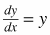3.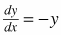4.5.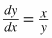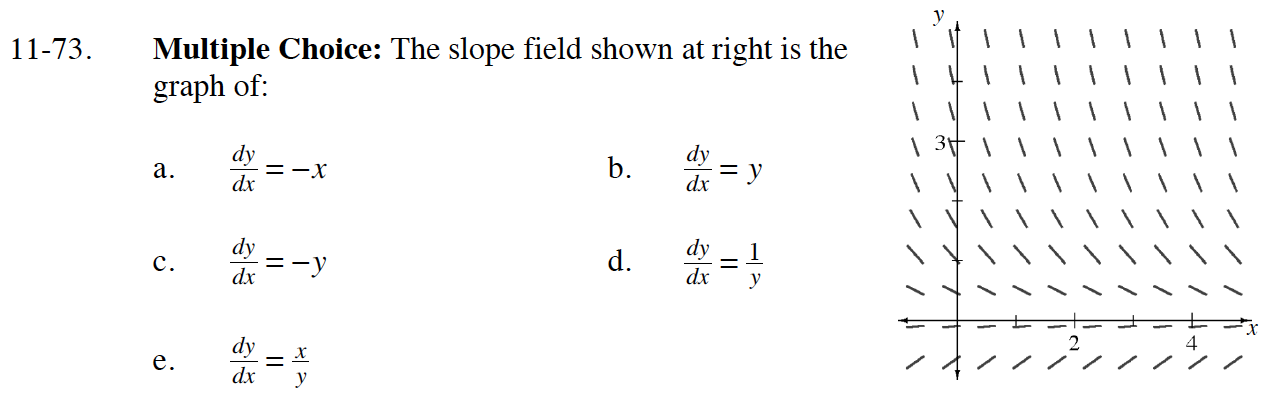When is the slope 0? Which equation could this match?

When are the slopes positive? When are the slopes negative?
Which equation could these situations match?